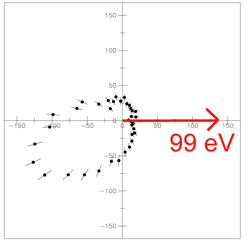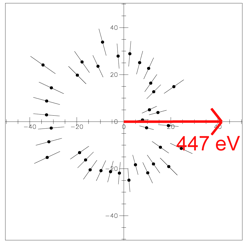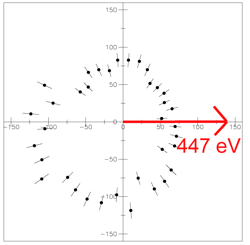﻿ Atomic Physics - photon-atom
 We have measured TDCS for the Photo Double Ionization of Helium at a photon energy of 179 eV. In the following angular distributions you see the circular dichroism.Figure 1: Electron distribution for left polarized light. The fast electron which has an energy of 99 eV is fixed by the red arrow. The TDCS of the slow electron is plotted.Figure 2: Electron distribution for right polarized light. Here the fast electron has an energy of 99 eV. The TDCS of the slow electron which has an energy of 1 eV is plotted. For left polarized light: The complementary electron which has an energy of 1 eV is emitted mostly into the upper hemisphere. In the case of the right polarized light the 1 eV electron is mostly emitted into the lower hemisphere. These angular distributions are a special case where the low energy electron has an energy of 1 eV. For a general view of the angular distributions: The movies show the 3 dimensional angular distributions of the two electrons in dependence of the energy of the slower electron. left polarized light (2.4 MB) right polarized light (2.4 MB) Note: The whole range of the energy of the slower electron is 50 eV. The "snakeline" is the circular polarized light, the faster electron is emitted perpendicular to the polarized light to the right side. What you see is the angular distribution of the complementary, the slower electron starting with an energy of 0 eV up to 50 eV of the slower electron. If we have a really slow electron you see a big difference in the angular distributions of the electrons for the case of left and right polarized light. The faster the slower electrons get the more you see that the circular dichroism vanishes. In the case of equal energy sharing you can't see any dichroism because of the indistinguishability of the two electrons. In that case you can't define a handeness. TDCS of Photo Double Ionization of Helium 450 eV above the threshold: We have measured TDCS for the Photo Double Ionization of Helium at a photon energy of 529 eV, as well.Figure 3: Left polarized light: the fast electron which has an energy of 447 eV is fixed by the red arrow. The TDCS of the slow electron is plotted.Figure 4: Right polarized light: here the fast electron has an energy of 447 eV. The TDCS of the slow electron which has an energy of 3 eV is plotted. For left polarized light: The complementary electron which has an energy of 3 eV is emitted mostly into the upper hemisphere. In the case of the right polarized light the 3 eV electron is mostly emitted into the lower hemisphere.